## How to calculate number of days in a month or a year in Excel?

As we all know, there are leap years and common years where leap year has 366 days and common year has 365 days. To calculate the number of days in a month or a year based on a date as below screenshot shown, this article will help you.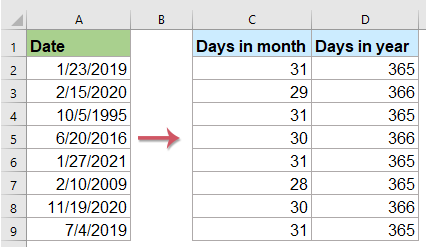#### Calculate number of days in a given month with formulas

If you have a list of date in a column, to calculate the days in a month, please apply the following formulas:

Enter any of the below formulas into a blank cell beside your date, and then drag the fill handle down to the cells that you want to calculate the days in the specific month, see screenshot:

=DAY(DATE(YEAR(A2),MONTH(A2)+1,1)-1)
=DAY(EOMONTH(A2,0))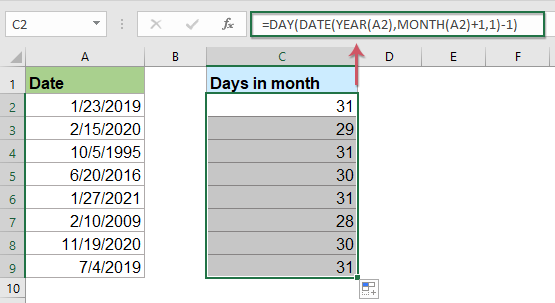Note: In the above formulas, A2 is the date cell that you want to get the number of days in a month based on.

#### Calculate number of days in a given year with formulas

To get the number of days in a year, please do as this:

Enter the following formula into a blank cell beside your date cell, then drag the fill handle down to the cells that you want to calculate the days in the specific year, see screenshot:

=DATE(YEAR(A2),12,31)-DATE(YEAR(A2),1,1)+1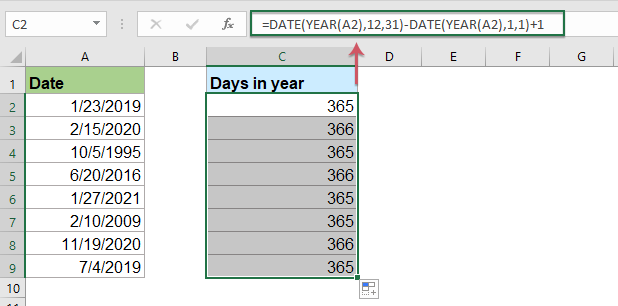Note: If you just have year numbers in the column, the below formula can help you to calculate the days in the given years as following screenshot shown:

=365+IF(MOD(A2,4),0,1)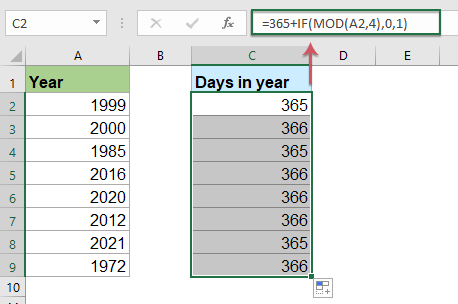#### Calculate number of days in a given month or year with a useful feature

If you have Kutools for Excel, with its Calculate days in year / Calculate days in month features, you can get the the number of days in a specific month or year without remembering any formulas.

Note:To apply these Calculate days in year / Calculate days in month features, firstly, you should download the Kutools for Excel, and then apply the features quickly and easily.

After installing Kutools for Excel, please do as follows:

1. Click to select a cell where you want to output the result, see screenshot: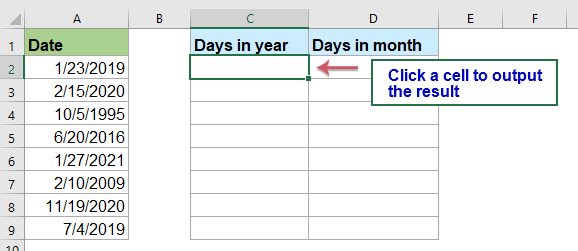2. And then click Kutools > Formula Helper > Formula Helper, see screenshot: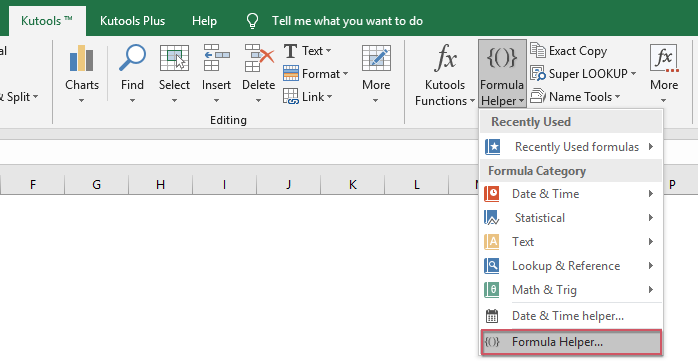3. In the Formulas Helper dialog box, please do the following operations:

• Select Math from the Formula Type drop down list;
• In the Choose a formula list box, click to select Calculate days in year or Calculate days in month option as you need;
• Then, in the Arguments input section, select the cell containing the date that you want to get the days of month or year from the date .

Tips: In the Date textbox, you should change the default absolute cell reference to relative cell reference for dragging the formula correctly.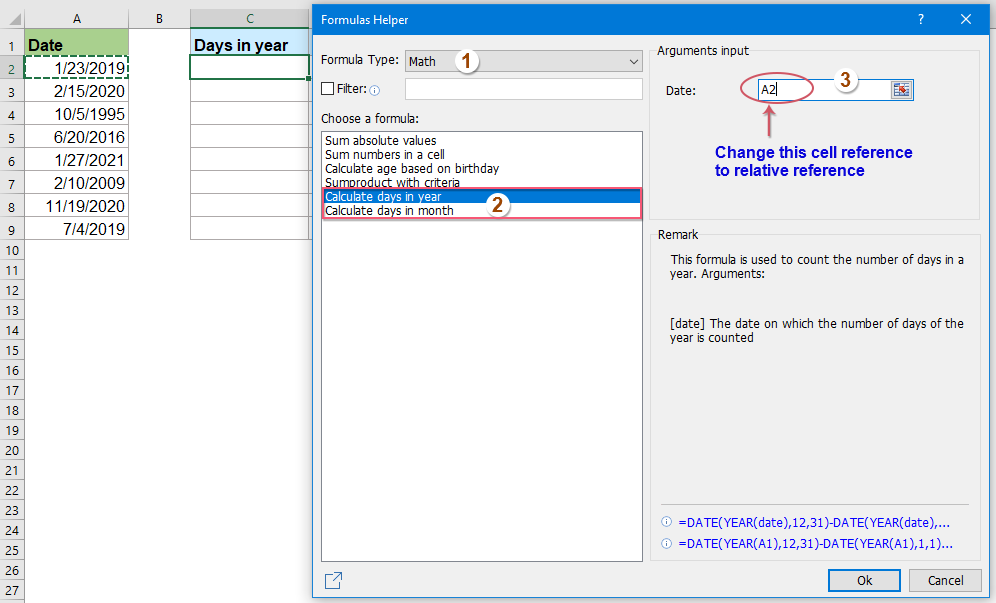4. And then, click Ok button, the first result will be calculated, then select the first formula cell, and drag the fill handle down to the cells for applying this formula, and you will get the number of days in a year as below screenshot shown: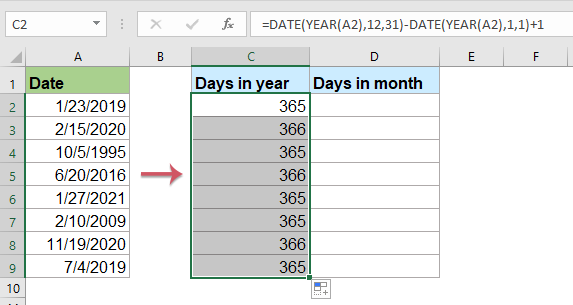Selecting the Calculate days in month option to get the number of days in specific months, see screenshot: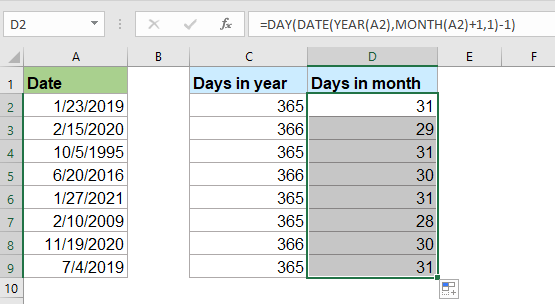#### More relative articles:

• Count The Number Of Days, Workdays, Weekends Between Two Dates
• Have you ever needed to count the number of days between two dates in Excel? May be, sometimes, you just only want to calculate the workdays between two dates, and sometime, you need to count the weekend days only between the two dates. How could you count the number of days between two dates in a certain condition?
• Calculate Days Left In Month Or Year In Excel
• For certain case, you may want to know the number of remaining days in a month or a year. Supposing, the date of today is 2014/10/12, and you want to calculate the days left in this month (October) or this year (2014), that is to say, there is remaining 19 days of this month and 80 days of this year. Please know more details from the below article.

### Best Office Productivity Tools

Supports Office/Excel 2007-2021 and 365  |  Available in 44 Languages  |  Easy to Uninstall Completely

 Popular Features: Find/Highlight/Identify Duplicates   |  Delete Blank Rows   |  Combine Columns or Cells without Losing Data   |   Round without Formula ... Super Lookup: Multiple Criteria VLookup  |   Multiple Value VLookup  |   VLookup Across Multiple Sheets   |   Fuzzy Lookup .... Advanced Drop-down List: Quickly Create Drop Down List   |  Dependent Drop Down List   |  Multi-select Drop Down List .... Column Manager: Add a Specific Number of Columns   |   Move Columns   |   Unhide Columns   |   Compare Columns to Select Same & Different Cells ... Featured Features: Grid Focus   |  Design View   |   Big Formula Bar   |  Workbook & Sheet Manager   |  Resource Library (Auto Text)   |  Date Picker   |  Combine Worksheets   |  Encrypt/Decrypt Cells   |  Send Emails by List   |  Super Filter   |   Special Filter (filter bold/italic/strikethrough...) ... Top 15 Toolset:  12 Text Tools (Add Text, Remove Characters, ...)   |   50+ Chart Types (Gantt Chart, ...)   |   40+ Practical Formulas (Calculate age based on birthday, ...)   |   19 Insertion Tools (Insert QR Code, Insert Picture from Path, ...)   |   12 Conversion Tools (Numbers to Words, Currency Conversion, ...)   |   7 Merge & Split Tools (Advanced Combine Rows, Split Cells, ...)   |   Many More...

Kutools for Excel Boasts Over 300 Features, Ensuring That What You Need Is Just A Click Away...

Supercharge Your Spreadsheets: Experience Efficiency Like Never Before with Kutools for Excel  (Full-Featured 30-Day Free Trial)#### Office Tab Brings Tabbed interface to Office, and Make Your Work Much Easier

• Enable tabbed editing and reading in Word, Excel, PowerPoint, Publisher, Access, Visio and Project.
• Open and create multiple documents in new tabs of the same window, rather than in new windows.
• Increases your productivity by 50%, and reduces hundreds of mouse clicks for you every day!No ratings yet. Be the first to rate!
This comment was minimized by the moderator on the site
Es ist wenig hilfreich, in einer deutschen Version der Seite die englischen Excel-Zelleinträge zu zeigen.
Insbesondere , und ; sind für deutschsprachige Nutzer (und dafür ist ja die Seite) kaum wahrzunehmen !
MfG
This comment was minimized by the moderator on the site
Your MONTH(EOMONTH(A2,(DAY(A2)>15)+0)) formula does not work correctly for 28, 29 or 30-day months due to the hard coded comparison to the 15th day of the month. For example, for the date 2/14/2021, it calculates the round-up month number to be 2 (February). But it should be 3 (March) given that the 14th is the half-month day of 28-day February (equivalent to a fraction value 0.5, which should round up, not down). Similarly, for the date 4/15/2021, it calculates the round-up month number to be 4 (April). But it should be 5 (May) given that the 15th is the half-month day of 30-day April (again equivalent to a fraction value 0.5).

Here's a corrected version of your formula that works for all months:

=MONTH(EOMONTH(A1,(DAY(A1)>=DAY(EOMONTH(A1,0))/2)-1)+1)
This comment was minimized by the moderator on the site
How about this for Number of Days in THIS year (covers leap year or not)…. =EOMONTH(DATE(YEAR(TODAY()),12,1),0) - DATE(YEAR(TODAY()),1,0)

EOMONTH finds December 31 of this year, from TODAY() and them subtracts the day before January 1st using the DATE YEAR TODAY using 0 which is one less than 1.
There are no comments posted here yet